# Birefringence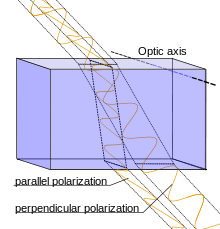Incoming light in the parallel (s) polarization sees a different effective index of refraction than light in the perpendicular (p) polarization, and is thus refracted at a different angle.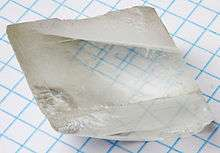A calcite crystal laid upon a graph paper with blue lines showing the double refraction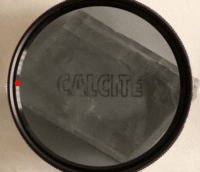Doubly refracted image as seen through a calcite crystal, seen through a rotating polarizing filter illustrating the opposite polarization states of the two images.

Birefringence is the optical property of a material having a refractive index that depends on the polarization and propagation direction of light. These optically anisotropic materials are said to be birefringent (or birefractive). The birefringence is often quantified as the maximum difference between refractive indices exhibited by the material. Crystals with non-cubic crystal structures are often birefringent, as are plastics under mechanical stress.

Birefringence is responsible for the phenomenon of double refraction whereby a ray of light, when incident upon a birefringent material, is split by polarization into two rays taking slightly different paths. This effect was first described by the Danish scientist Rasmus Bartholin in 1669, who observed it in calcite, a crystal having one of the strongest birefringences. However it was not until the 19th century that Augustin-Jean Fresnel described the phenomenon in terms of polarization, understanding light as a wave with field components in transverse polarizations (perpendicular to the direction of the wave vector).

## Explanation

A mathematical description of wave propagation in a birefringent medium is presented below. Following is a qualitative explanation of the phenomenon.

### Uniaxial materials

The simplest (and most common) type of birefringence is described as uniaxial, meaning that there is a single direction governing the optical anisotropy whereas all directions perpendicular to it (or at a given angle to it) are optically equivalent. Thus rotating the material around this axis does not change its optical behavior. This special direction is known as the optic axis of the material. Light whose polarization is perpendicular to the optic axis is governed by a refractive index no (for "ordinary"). Light whose polarization is in the direction of the optic axis sees an optical index ne (for "extraordinary"). For any ray direction there is a linear polarization direction perpendicular to the optic axis, and this is called an ordinary ray. However, for ray directions not parallel to the optic axis, the polarization direction perpendicular to the ordinary ray's polarization will be partly in the direction of the optic axis, and this is called an extraordinary ray. The ordinary ray will always experience a refractive index of no, whereas the refractive index of the extraordinary ray will be in between no and ne, depending on the ray direction as described by the index ellipsoid. The magnitude of the difference is quantified by the birefringence:.

The propagation (as well as reflection coefficient) of the ordinary ray is simply described by no as if there were no birefringence involved. However the extraordinary ray, as its name suggests, propagates unlike any wave in a homogenous optical material. Its refraction (and reflection) at a surface can be understood using the effective refractive index (a value in between no and ne). However it is in fact an inhomogeneous wave whose power flow (given by the Poynting vector) is not exactly in the direction of the wave vector. This causes an additional shift in that beam, even when launched at normal incidence, as is popularly observed using a crystal of calcite as photographed above. Rotating the calcite crystal will cause one of the two images, that of the extraordinary ray, to rotate slightly around that of the ordinary ray, which remains fixed.

When the light propagates either along or orthogonal to the optic axis, such a lateral shift does not occur. In the first case, both polarizations see the same effective refractive index, so there is no extraordinary ray. In the second case the extraordinary ray propagates at a different phase velocity (corresponding to ne) but is not an inhomogeneous wave. A crystal with its optic axis in this orientation, parallel to the optical surface, may be used to create a waveplate, in which there is no distortion of the image but an intentional modification of the state of polarization of the incident wave. For instance, a quarter-wave plate is commonly used to create circular polarization from a linearly polarized source.

### Biaxial materials

The case of so-called biaxial crystals is substantially more complex. These are characterized by three refractive indices corresponding to three principal axes of the crystal. For most ray directions, both polarizations would be classified as extraordinary rays but with different effective refractive indices. Being extraordinary waves, however, the direction of power flow is not identical to the direction of the wave vector in either case.

The two refractive indices can be determined using the index ellipsoids for given directions of the polarization. Note that for biaxial crystals the index ellipsoid will not be an ellipsoid of revolution ("spheroid") but is described by three unequal principle refractive indices nα, nβ and nγ. Thus there is no axis around which a rotation leaves the optical properties invariant (as there is with uniaxial crystals whose index ellipsoid is a spheroid).

Although there is no axis of symmetry, there are two optical axes or binormals which are defined as directions along which light may propagate without birefringence, i.e., directions along which the wavelength is independent of polarization. For this reason, birefringent materials with three distinct refractive indices are called biaxial. Additionally, there are two distinct axes known as optical ray axes or biradials along which the group velocity of the light is independent of polarization.

### Double refraction

When an arbitrary beam of light strikes the surface of a birefringent material, the polarizations corresponding to the ordinary and extraordinary rays generally take somewhat different paths. Unpolarized light consists of equal amounts of energy in any two orthogonal polarizations, and even polarized light (except in special cases) will have some energy in each of these polarizations. According to Snell's law of refraction, the angle of refraction will be governed by the effective refractive index which is different between these two polarizations. This is clearly seen, for instance, in the Wollaston prism which is designed to separate incoming light into two linear polarizations using a birefringent material such as calcite.

The different angles of refraction for the two polarization components are shown in the figure at the top of the page, with the optic axis along the surface (and perpendicular to the plane of incidence), so that the angle of refraction is different for the p polarization (the "ordinary ray" in this case, having its polarization perpendicular to the optic axis) and the s polarization (the "extraordinary ray" with a polarization component along the optic axis). In addition, a distinct form of double refraction occurs in cases where the optic axis is not along the refracting surface (nor exactly normal to it); in this case the electric polarization of the birefringent material is not exactly in the direction of the wave's electric field for the extraordinary ray. The direction of power flow (given by the Poynting vector) for this inhomogenous wave is at a finite angle from the direction of the wave vector resulting in an additional separation between these beams. So even in the case of normal incidence, where the angle of refraction is zero (according to Snell's law, regardless of effective index of refraction), the energy of the extraordinary ray may be propagated at an angle. This is commonly observed using a piece of calcite cut appropriately with respect to its optic axis, placed above a paper with writing, as in the above two photographs.

## Terminology

Much of the work involving polarization preceded the understanding of light as a transverse electromagnetic wave, and this has affected some terminology in use. Isotropic materials have symmetry in all directions and the refractive index is the same for any polarization direction. An anisotropic material is called "birefringent" because it will generally refract a single incoming ray in two directions, which we now understand correspond to the two different polarizations. This is true of either a uniaxial or biaxial material.

In a uniaxial material, one ray behaves according to the normal law of refraction (corresponding to the ordinary refractive index), so an incoming ray at normal incidence remains normal to the refracting surface. However, as explained above, the other polarization can be deviated from normal incidence, which cannot be described using the law of refraction. This thus became known as the extraordinary ray. The terms "ordinary" and "extraordinary" are still applied to the polarization components perpendicular to and not perpendicular to the optic axis respectively, even in cases where no double refraction is involved.

A material is termed uniaxial when it has a single direction of symmetry in its optical behavior, which we term the optic axis. It also happens to be the axis of symmetry of the index ellipsoid (a spheroid in this case). The index ellipsoid could still be described according to the refractive indices, nα, nβ and nγ, along three coordinate axes, however in this case two are equal. So if nα = nβ corresponding to the x and y axes, then the extraordinary index is nγ corresponding to the z axis, which is also called the optic axis in this case.

However materials in which all three refractive indices are different are termed biaxial and the origin of this term is more complicated and frequently misunderstood. In a uniaxial crystal, different polarization components of a beam will travel at different phase velocities, except for rays in the direction of what we call the optic axis. Thus the optic axis has the particular property that rays in that direction do not exhibit birefringence, with all polarizations in such a beam experiencing the same index of refraction. It is very different when the three principal refractive indices are all different; then an incoming ray in any of those principle directions will still encounter two different refractive indices. But it turns out that there are two special directions (at an angle to all of the 3 axes) where the refractive indices for different polarizations are again equal. For this reason, these crystals were designated as biaxial, with the two "axes" in this case referring to ray directions in which propagation does not experience birefringence.

### Fast and slow rays

In a birefringent material, a wave consists of two polarization components which generally are governed by different effective refractive indices. The so-called slow ray is the component for which the material has the higher effective refractive index (slower phase velocity), while the fast ray is the one with a lower effective refractive index. When a beam is incident on such a material from air (or any material with a lower refractive index), the slow ray is thus refracted more towards the normal than the fast ray. In the figure at the top of the page, it can be seen that refracted ray with s polarization in the direction of the optic axis (thus the extraordinary ray) is the slow ray in this case.

Using a thin slab of that material at normal incidence, one would implement a waveplate. In this case there is essentially no spatial separation between the polarizations, however the phase of the wave in the parallel polarization (the slow ray) will be retarded with respect to the perpendicular polarization. These directions are thus known as the slow axis and fast axis of the waveplate.

### Positive or negative

Uniaxial birefringence is classified as positive when the extraordinary index of refraction ne is greater than the ordinary index no. Negative birefringence means that Δn = neno is less than zero. In other words, the polarization of the fast (or slow) wave is perpendicular to the optic axis when the birefringence of the crystal is positive (or negative, respectively). In the case of biaxial crystals, all three of the principal axes have different refractive indices, so this designation does not apply. But for any defined ray direction one can just as well designate the fast and slow ray polarizations.

## Sources of optical birefringence

While birefringence is usually obtained using an anisotropic crystal, it can result from an optically isotropic material in a few ways:

• Stress birefringence results when isotropic materials are stressed or deformed (i.e., stretched or bent) causing a loss of physical isotropy and consequently a loss of isotropy in the material's permittivity tensor.
• Circular birefringence in liquids where there is a enantiomeric excess in a solution containing a molecule which has stereo isomers.
• Form birefringence, whereby structure elements such as rods, having one refractive index, are suspended in a medium with a different refractive index. When the lattice spacing is much smaller than a wavelength, such a structure is described as a metamaterial.
• By the Kerr effect, whereby an applied electric field induces birefringence at optical frequencies through the effect of nonlinear optics;
• By the Faraday effect, where a magnetic field causes some materials to become circularly birefringent (having slightly different indices of refraction for left and right handed circular polarizations), making the material optically active until the field is removed;
• By the self or forced alignment into thin films of amphiphilic molecules such as lipids, some surfactants or liquid crystals

## Common birefringent materials

The best characterized birefringent materials are crystals. Due to their specific crystal structures their refractive indices are well defined. Depending on the symmetry of a crystal structure (as determined by one of the 32 possible crystallographic point groups), crystals in that group may be forced to be isotropic (not birefringent), to have uniaxial symmetry, or neither in which case it is a biaxial crystal. The crystal structures permitting uniaxial and biaxial birefringence are noted in the two tables, below, listing the two or three principal refractive indices (at wavelength 590 nm) of some better known crystals.

Many plastics are birefringent because their molecules are "frozen" in a stretched conformation when the plastic is molded or extruded. For example, ordinary cellophane is birefringent. Polarizers are routinely used to detect stress in plastics such as polystyrene and polycarbonate.

Cotton fiber is birefringent because of high levels of cellulosic material in the fiber's secondary cell wall.

Polarized light microscopy is commonly used in biological tissue, as many biological materials are birefringent. Collagen, found in cartilage, tendon, bone, corneas, and several other areas in the body, is birefringent and commonly studied with polarized light microscopy. Some proteins are also birefringent, exhibiting form birefringence.

Inevitable manufacturing imperfections in optical fiber leads to birefringence, which is one cause of pulse broadening in fiber-optic communications. Such imperfections can be geometrical (lack of circular symmetry), due to stress applied to the optical fiber and/or due to bending of the fiber. Birefringence is intentionally introduced (for instance, by making the cross-section elliptical) in order to produce polarization-maintaining optical fibers.

In addition to anisotropy in the electric polarizability (electric susceptibility), anisotropy in the magnetic polarizability (magnetic permeability) can also cause birefringence. However, at optical frequencies, values of magnetic permeability for natural materials are not measurably different from µ0, so this is not a source of optical birefringence in practice.

Uniaxial crystals, at 590 nm
Material Crystal system no ne Δn
barium borate BaB2O4 Trigonal 1.6776 1.5534 −0.1242
beryl Be3Al2(SiO3)6 Hexagonal 1.602 1.557 −0.045
calcite CaCO3 Trigonal 1.658 1.486 −0.172
ice H2O Hexagonal 1.309 1.313 +0.004
lithium niobate LiNbO3 Trigonal 2.272 2.187 −0.085
magnesium fluoride MgF2 Tetragonal 1.380 1.385 +0.006
quartz SiO2 Trigonal 1.544 1.553 +0.009
ruby Al2O3 Trigonal 1.770 1.762 −0.008
rutile TiO2 Tetragonal 2.616 2.903 +0.287
sapphire Al2O3 Trigonal 1.768 1.760 −0.008
silicon carbide SiC Hexagonal 2.647 2.693 +0.046
tourmaline (complex silicate ) Trigonal 1.669 1.638 −0.031
zircon, high ZrSiO4 Tetragonal 1.960 2.015 +0.055
zircon, low ZrSiO4 Tetragonal 1.920 1.967 +0.047
Biaxial crystals, at 590 nm
Material Crystal system nα nβ nγ
borax Na2(B4O5)(OH)4·8(H2O) Monoclinic1.447 1.469 1.472
epsom salt MgSO4·7(H2O) Monoclinic1.433 1.455 1.461
mica, biotite K(Mg,Fe)
3
AlSi
3
O
10
(F,OH)
2
Monoclinic 1.595 1.640 1.640
mica, muscovite KAl2(AlSi3O10)(F,OH)2 Monoclinic 1.563 1.596 1.601
olivine (Mg, Fe)2SiO4Orthorhombic1.640 1.660 1.680
perovskite CaTiO3 Orthorhombic2.300 2.340 2.380
topaz Al2SiO4(F,OH)2 Orthorhombic1.618 1.620 1.627
ulexite NaCaB5O6(OH)6·5(H2O) Triclinic1.490 1.510 1.520

## Measurement

Birefringence and other polarization-based optical effects (such as optical rotation and linear or circular dichroism) can be measured by measuring the changes in the polarization of light passing through the material. These measurements are known as polarimetry. Polarized light microscopes, which contain two polarizers that are at 90° to each other on either side of the sample, are used to visualize birefringence. The addition of quarter-wave plates permit examination of circularly polarized light. Birefringence measurements have been made with phase-modulated systems for examining the transient flow behavior of fluids.

Birefringence of lipid bilayers can be measured using dual polarization interferometry. This provides a measure of the degree of order within these fluid layers and how this order is disrupted when the layer interacts with other biomolecules.

## Applications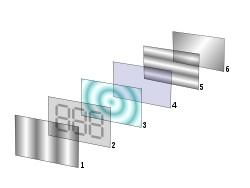Reflective twisted-nematic liquid-crystal display. Light reflected by surface (6) (or coming from a backlight) is horizontally polarized (5) and passes through the liquid-crystal modulator (3) sandwiched in between transparent layers (2, 4) containing electrodes. Horizontally polarized light is blocked by the vertically oriented polarizer (1), except where its polarization has been rotated by the liquid crystal (3), appearing bright to the viewer.

Birefringence is used in many optical devices. Liquid-crystal displays, the most common sort of flat panel display, cause their pixels to become lighter or darker through rotation of the polarization (circular birefringence) of linearly polarized light as viewed through a sheet polarizer at the screen's surface. Similarly, light modulators modulate the intensity of light through electrically induced birefringence of polarized light followed by a polarizer. The Lyot filter is a specialized narrowband spectral filter employing the wavelength dependence of birefringence. Wave plates are thin birefringent sheets widely used in certain optical equipment for modifying the polarization state of light passing through it.

Birefringence also plays an important role in second-harmonic generation and other nonlinear optical components, as the crystals used for this purpose are almost always birefringent. By adjusting the angle of incidence, the effective refractive index of the extraordinary ray can be tuned in order to achieve phase matching, which is required for efficient operation of these devices.

### Medicine

Birefringence is utilized in medical diagnostics. One powerful accessory used with optical microscopes is a pair of crossed polarizing filters. Light from the source is polarized in the X direction after passing through the first polarizer, but above the specimen is a polarizer (a so-called analyzer) oriented in the Y direction. Therefore, no light from the source will be accepted by the analyzer, and the field will appear dark. However areas of the sample possessing birefringence will generally couple some of the X-polarized light into the Y polarization; these areas will then appear bright against the dark background. Modifications to this basic principle can differentiate between positive and negative birefringence.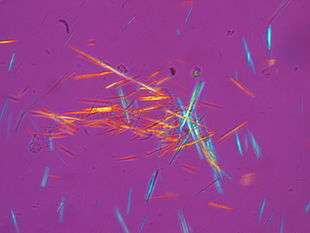Urate crystals, with the crystals with their long axis seen as horizontal in this view being parallel to that of a red compensator filter. These appear as yellow, and are thereby of negative birefringence.

For instance, needle aspiration of fluid from a gouty joint will reveal negatively birefringent monosodium urate crystals. Calcium pyrophosphate crystals, in contrast, show weak positive birefringence. Urate crystals appear yellow, and calcium pyrophosphate crystals appear blue when their long axes are aligned parallel to that of a red compensator filter, or a crystal of known birefringence is added to the sample for comparison.

Birefringence can be observed in amyloid plaques such as are found in the brains of Alzheimer's patients when stained with a dye such as Congo Red. Modified proteins such as immunoglobulin light chains abnormally accumulate between cells, forming fibrils. Multiple folds of these fibers line up and take on a beta-pleated sheet conformation. Congo red dye intercalates between the folds and, when observed under polarized light, causes birefringence.

In ophthalmology, binocular retinal birefringence screening of the Henle fibers (photoreceptor axons that go radially outward from the fovea) provides a reliable detection of strabismus and possibly also of anisometropic amblyopia. Furthermore, scanning laser polarimetry utilises the birefringence of the optic nerve fibre layer to indirectly quantify its thickness, which is of use in the assessment and monitoring of glaucoma.

Birefringence characteristics in sperm heads allow the selection of spermatozoa for intracytoplasmic sperm injection. Likewise, zona imaging uses birefringence on oocytes to select the ones with highest chances of successful pregnancy. Birefringence of particles biopsied from pulmonary nodules indicates silicosis.

Dermatologists use dermatascopes to view pigmented lesions and nevi. Dermatascopes use cross-polarized light, allowing the user to view chrystaline structures corresponding to dermal collagen in the skin. These structures may appear as shiny white lines or rosette shapes and are only visible under polarized dermoscopy.

### Stress-induced birefringenceColor pattern of a plastic box with "frozen in" mechanical stress placed between two crossed polarizers

Isotropic solids do not exhibit birefringence. However, when they are under mechanical stress, birefringence results. The stress can be applied externally or is "frozen in" after a birefringent plastic ware is cooled after it is manufactured using injection molding. When such a sample is placed between two crossed polarizers, colour patterns can be observed, because polarization of a light ray is rotated after passing through a birefingent material and the amount of rotation is dependent on wavelength. The experimental method called photoelasticity used for analyzing stress distribution in solids is based on the same principle.

### Other cases of birefringence

Birefringence is observed in anisotropic elastic materials. In these materials, the two polarizations split according to their effective refractive indices, which are also sensitive to stress.

The study of birefringence in shear waves traveling through the solid Earth (the Earth's liquid core does not support shear waves) is widely used in seismology.

Birefringence is widely used in mineralogy to identify rocks, minerals, and gemstones.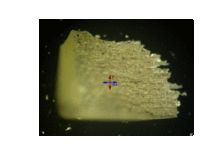Birefringent rutile observed in different polarizations using a rotating polarizer (or analyzer)

## Theory

In an isotropic medium (including free space) the so-called electric displacement (D) is just proportional to the electric field (E) according towhere the material's permittivity ε is just a scalar (and equal to the square of the index of refraction n times ε0). However, in an anisotropic material exhibiting birefringence, the relationship between D and E must now be described using a tensor equation:where ε is now a 3x3 permittivity tensor. We assume linearity and no magnetic permeability in the medium:. The electric field of a plane wave of angular frequency ω can be written in the general form:(2)

where r is the position vector, t is time, and E0 is a vector describing the electric field at r=0, t=0. Then we shall find the possible wave vectors k. By combining Maxwell's equations forand, we can eliminateto obtain:(3a)

With no free charges, Maxwell's equation for the divergence of D vanishes:(3b)

We can apply the vector identity:to the left hand side of eqn. 3a, and use the spatial dependence in which each differentiation in x (for instance) results in multiplication byto find:.

The right hand side of eqn. 3a can be expressed in terms of E through application of the permitivity tensor ε and noting that differentiation in time results in multiplication by, eqn. 3a then becomes:(4a)

Applying the differentiation rule to eqn. 3b we find:(4b)

Eqn. 4b indicates thatis orthogonal to the direction of the wavevector k, even though that is no longer generally true foras would be the case in an isotropic medium. Eqn. 4b will not be needed for the further steps in the following derivation.

Finding the allowed values of k for a given ω is easiest done by using Cartesian coordinates with the x, y and z axes chosen in the directions of the symmetry axes of the crystal (or simply choosing z in the direction of the optic axis of a uniaxial crystal), resulting in a diagonal matrix for the permitivity tensor ε:(4c)

where the diagonal values are squares of the refractive indices for polarizations along the three principal axes x, y and z. With ε in this form, and substituting in the speed of light c using, eqn. 4a becomes(5a)

where Ex, Ey, Ez are the components of E (at any given position in space and time) and kx, ky and kz are the components of k. Rearranging, we can write (and similarly for the y and z components of eqn. 4a):(5b)(5c)(5d)

This is a set of linear equations in Ex, Ey, Ez, so it can have a non-trivial solution (that is, one other than E=0) as long as the following determinant is zero:(6)

Evaluating the determinant of eqn (6), and rearranging the terms, we obtainIn the case of a uniaxial material, choosing the optic axis to be in the z direction so that nx=ny=no and nz=ne, this expression can be factored into(8)

Setting either of the factors in equation (8) to zero will define an ellipsoidal surface[note 1] in the space of wave vectors k that are allowed for a given ω. The first factor being zero defines a sphere; this is the solution for "ordinary rays", in which the effective refractive index is exactly no regardless of the direction of k. The second defines a spheroid symmetric about the z axis. This solution corresponds to the "extraordinary rays" in which the effective refractive index is in between no and ne, depending on the direction of k. Therefore, for any arbitrary direction of propagation (other than in the direction of the optic axis), two distinct wavevectors k are allowed corresponding to the polarizations of the ordinary and extraordinary rays.

For a biaxial material a similar but more complicated condition on the two waves can be described; the allowed k vectors in a specified direction now lie on one of two ellipsoids. By inspection one can see that eqn. 6 is generally satisfied for two positive values of ω. Or for a specified optical frequency ω and direction normal to the wavefronts k/|k|, it is satisfied for two wavenumbers (propagation constants) |k| (and thus effective refractive indices) corresponding to the propagation of two linear polarizations in that direction.

When those two propagation constants are equal then the effective refractive index is independent of polarization, and there is consequently no birefringence encountered by a wave traveling in that particular direction. For a uniaxial crystal, this is the optic axis, the z direction according to the above construction. But when all three refractive indices (or permittivities), nx, ny and nz are distinct, it can be shown that there are exactly two such directions (where the two ellipsoids intersect); these directions are not at all obvious and do not lie along any of the three principal axes (x, y, and z according to the above convention). Historically that accounts for the use of the term "biaxial" for such crystals, as the existence of exactly two such special directions (considered "axes") was discovered well before polarization and birefringence were understood physically. However these two special directions are generally not of particular interest; biaxial crystals are rather specified by their three refractive indices corresponding to the three axes of symmetry.

A general state of polarization launched into the medium can always be decomposed into two waves, one in each of those two polarizations, which will then propagate with different wavenumbers |k|. Applying the different phase of propagation to those two waves over a specified propagation distance will result in a generally different net polarization state at that point; this is the principle of the waveplate for instance. However, when you have a wave launched into a birefringent material at non-normal incidence, the problem is yet more complicated since the two polarization components will now not only have distinct wavenumbers but the k vectors will not even be in exactly the same direction (see figure at the top of the page). In this case the two k vectors are rather solutions of eqn. 6 constrained by the boundary condition which requires that the components of the two transmitted waves' k vectors, and the k vector of the incident wave, as projected onto the surface of the interface must all be identical.

## Notes

1. Although related, note that this is not the same as the index ellipsoid

## References

1. "Olympus Microscopy Resource Center". Olympus America Inc. Retrieved 2011-11-13.
2. See:
3. Landau, L. D., and Lifshitz, E. M., Electrodynamics of Continuous Media, Vol. 8 of the Course of Theoretical Physics 1960 (Pergamon Press), §79
4. Brad Amos. Birefringence for facetors I: what is birefringence? Archived December 14, 2013, at the Wayback Machine. First published in StoneChat, the Journal of the UK Facet Cutter's Guild. January–March. edition 2005.
5. Elert, Glenn. "Refraction". The Physics Hypertextbook.
6. M. Wolman, F. H. Kasten, "Polarized light microscopy in the study of the molecular structure of collagen and reticulin", Histochemistry (1986), 85:41–49.
7. Sano, Y. "Optical anistropy of bovine serum albumin", J. Colliod Int. Sci., 124: 403–407 (1988).
8. Frattini, P., Fuller, G., "A note on phase-modulated flow birefringence: a promising rheo-optical method", J. Rheol., 28: 61 (1984).
9. Doyle, P., Shaqfeh, E. S. G., Spiegelberg, S. H., McKinley, G. H., "Relaxation of dilute polymer solutions following extensional flow", J. Non-Newtonian Fluid Mech., 86:79–110 (1998).
10. Hardy RH, Nation B (June 1984). "Acute gout and the accident and emergency department". Arch Emerg Med. 1 (2): 89–95. doi:10.1136/emj.1.2.89. PMC 1285204. PMID 6536274.
11. The Approach to the Painful Joint Workup Author: Alan N. Baer; Chief Editor: Herbert S. Diamond. Updated: Nov 22, 2010.
12. Reed M. Jost; Joost Felius; Eileen E. Birch (August 2014). "High sensitivity of binocular retinal birefringence screening for anisometropic amblyopia without strabismus". Journal of American Association for Pediatric Ophthalmology and Strabismus (JAAPOS). 18 (4): e5–e6.
13. Gianaroli L.; Magli M. C.; Ferraretti A. P.; et al. (December 2008). "Birefringence characteristics in sperm heads allow for the selection of reacted spermatozoa for intracytoplasmic sperm injection". Fertil. Steril. 93 (3): 807–13. doi:10.1016/j.fertnstert.2008.10.024. PMID 19064263.
14. Ebner T.; Balaban B.; Moser M.; et al. (May 2009). "Automatic user-independent zona pellucida imaging at the oocyte stage allows for the prediction of preimplantation development". Fertil. Steril. 94 (3): 913–920. doi:10.1016/j.fertnstert.2009.03.106. PMID 19439291.
15. Born M, and Wolf E, Principles of Optics, 7th Ed. 1999 (Cambridge University Press), §15.3.3Wikimedia Commons has media related to Birefringence.